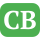CppBuzz.com

# Java Problems on Array for Beginners and Experts

These problems are very useful to improve your Java programming. There are various ways to solved these Java problem but suggestion is to write readable and customized code. Eclipse IDE can be used for writing & compilation of code.

Problem 1. Write a Java program that calculate sum of all diagonal elements of 4*4 double dimensional array. Output should be 36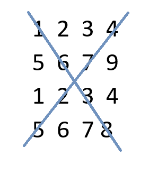Output: 36

Problem 2. Write a Java program to find sum of even and odd number separately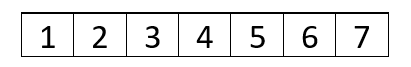Output should be:
Even: 12
Odd: 16

Problem 3. Find the length of integer array without using any predefined functionOutput: 7

Problem 4. Create two arrays with same no of integer element and create third array which contains sum of two arrays
Array1: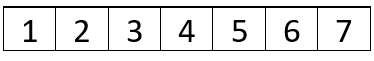Array2:Output: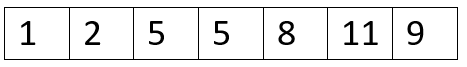Problem 5. Write Java code to check if two Integer arrays are identical
Array1:Array2:Output: Array are Identical

Problem 6. Write Java code to transpose two dimensional array. Program should be able to transpose n*n size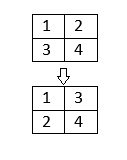Problem 7. Write Java code to convert integer [][] to integer[]. Code should support n number of elements.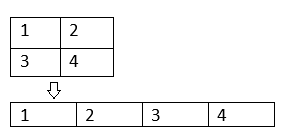Problem 8. Write Java code to reverse a SDM without using any predefined method of Java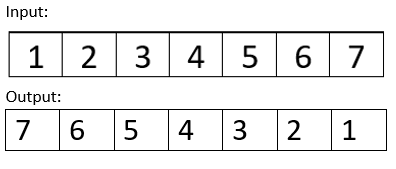Problem 9. Write Java program to short one array using index values which are in another array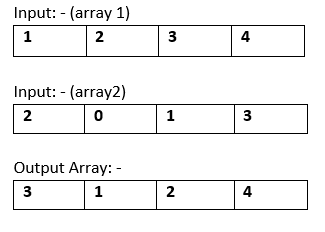Problem 10. Write Java code to find regions of connected 1’s in DDM (m*n) which is filled of 0 and 1. Here is an example Example 1:-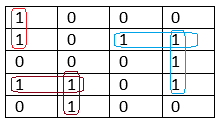Answer: Regions of Regions of connected 1s are: 3

Example 2:-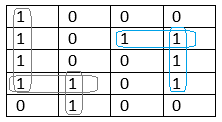Answer: Regions of Regions of connected 1s are: 2

Example 3:-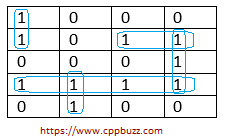Answer: Regions of Regions of connected 1s are: 2